#### You may also like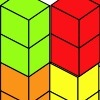### Triple Cubes

This challenge involves eight three-cube models made from interlocking cubes. Investigate different ways of putting the models together then compare your constructions.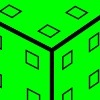### Inky Cube

This cube has ink on each face which leaves marks on paper as it is rolled. Can you work out what is on each face and the route it has taken?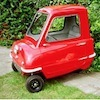### The Car That Passes

What statements can you make about the car that passes the school gates at 11am on Monday? How will you come up with statements and test your ideas?

##### Age 7 to 11Challenge Level

This is your chance to follow some numbers and see where they go!

A simple rule is all you need.
My first suggestion is to add the digits together then multiply (times) by $2$.
The first number that I chose happened to be $56$.

So, let's start:

We add the $5$ and $6$, $5+6=11$
We multiply the $11$ by $2$, $2 \times 11 = 22$, and that's the first part of the journey.

So, where now?
We carry on with this rule:

We add the $2$ and $2$,  $2+2=4$
We multiply the $4$ by $2$,  $4 \times 2 = 8$, and that's the second part the journey.

Now, $8 + 0 = 8$ and $8 \times 2 = 16$ and that was the third part.

And, $1 + 6= 7$, and $7 \times 2 = 14$

Do the same to $14$ and we get $10$

$10$ leads to $2$, $2$ leads to $4$, $4$ leads to $8$ and we are back to where we got to in the second part of the journey.

If we went on and on and wrote down where we got to after each part we would see something like:
$56, 22, 8, 16, 14, 10, 2, 4, 8, 16, 14, 10, 2, 4, 8, ...$

After exploring that journey it's time to start somewhere new, for example $11$ which goes along like this;:

$11, 4, 8, 16, 14, 10, 2, 4, 8, ...$

Oh! So we are on the same bit as before, a circular bit that goes $2, 4, 8, 16, 14, 10$ and then back to the $2$ again.

Now a new starting place, $96$.
This goes like this:

$96, 30, 6, 12, 6, 12, 6, ...$

Oh! So we now have a smaller circular bit of the journey that goes $6, 12$ then back to the $6$.

I explored further trying to start with each number from $1$ to $99$.

Then I tried similar, but different rules.
I found I needed a big piece of plain paper and used arrows to show the journeys.  Here are just some bits of them to tempt you to go further: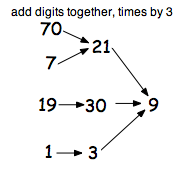and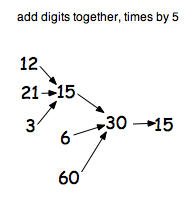There are $99$ starting points to try and I've only show you $8$ on each of the two above so there are lots more to explore!

Decide on the rules you will use and investigate what happens with different starting points.

We'd love to hear how you got on.

### Why do this problem?

This activity is one which is accessible to a very wide range of children. It also opens out the possibility of pupils asking “I wonder what would happen if . . .?” Pupils' curiosity may be easily aroused while finding exploring further. It enables them to enjoy 'playing with' numbers, and discover  peculiar yet interesting things that can happen! It could be used to raise some pupils' self esteem in mathematics, whilst  still giving scope for others to explore further and further showing their resilience and perseverance.

### Possible approach

Ask pupils to choose a number then tell them the  rule:  add the digits and multiply by two.  Follow the rule a few times so that the children are happy with it. For some pupils that act of suggesting the starting number helps them  feel able to participate. Once the rule has been applied then of course you could try a new starting number.

Give children the chance to work in pairs or small groups.   Introducing the need to record what you get could be approached  after a short time so that "numbers are not lost"!

This activity may be suitable for 'simmering' over a period of several days or weeks.  You could dedicate some wall space to it so children could post up their 'journeys' for particular starting numbers. It could then become a whole class investigation.

### Key questions

Whilst they are working on their own or in small groups you can ask them to tell you anything that they notice.
Can you find attractive ways of displaying their numbers for others to see?
What would you like to call this group of numbers that you've discovered?
Have you got to an end? If so how do you know?
What could you try now that you seem to have got to the end of that journey of numbers?

### Possible extension

Make sure that every number from $1$ to $99$ has been used to start the journeys, sticking to the same rule. Pupils should be able to ask themselves "I wonder what would happen if I ...?", so they can change one part of the rule.
When two or more have been completed these can then be compared and pupils can be encouraged to make predictions about future results.

### Possible support

Plenty of digit cards will help children who find recording more difficult.

### For the highest-attaining

When quite a few have been completed  encourage pupils to compare them carefully.  What is similar, different and the same?  Some might like to show the sequences using their choice of appropriate software. They could then derive hypotheses relating to the sequences and test them.